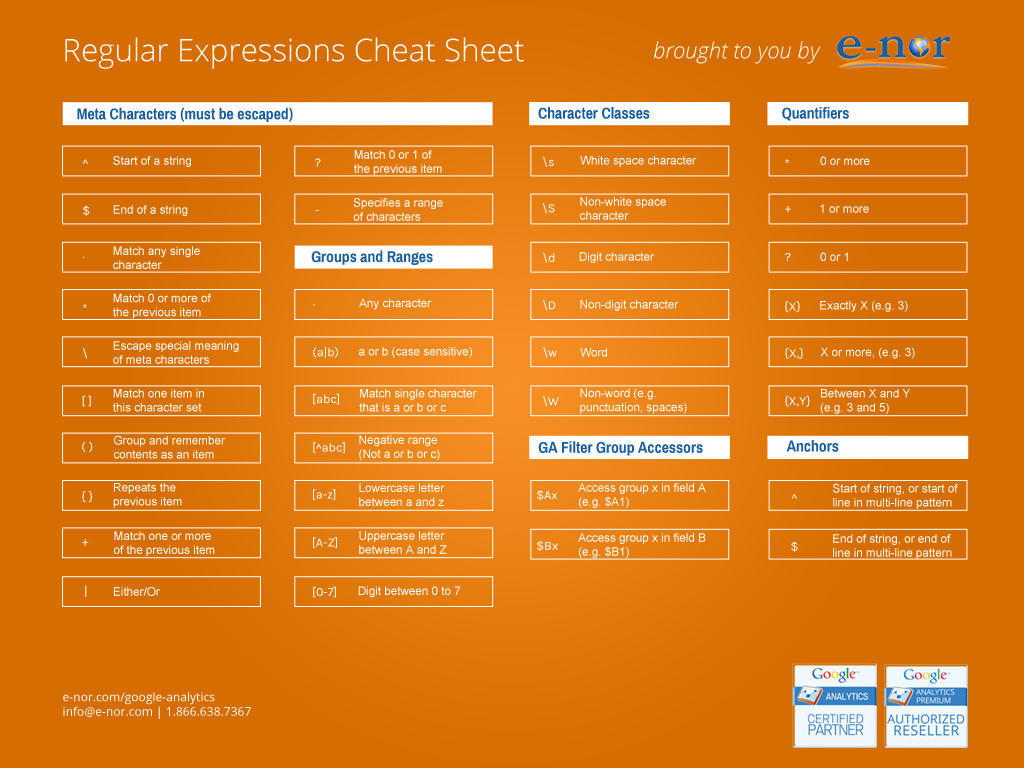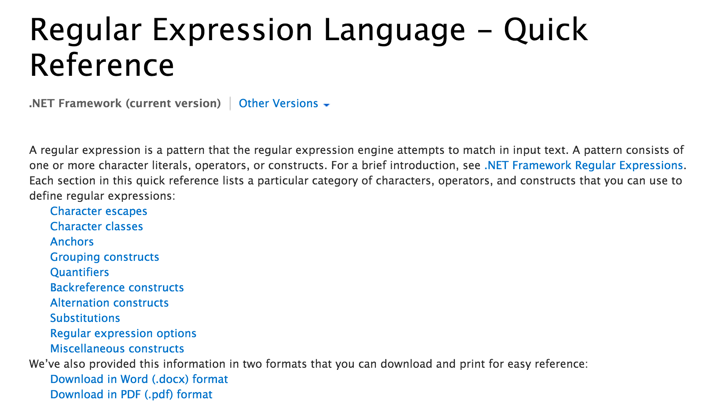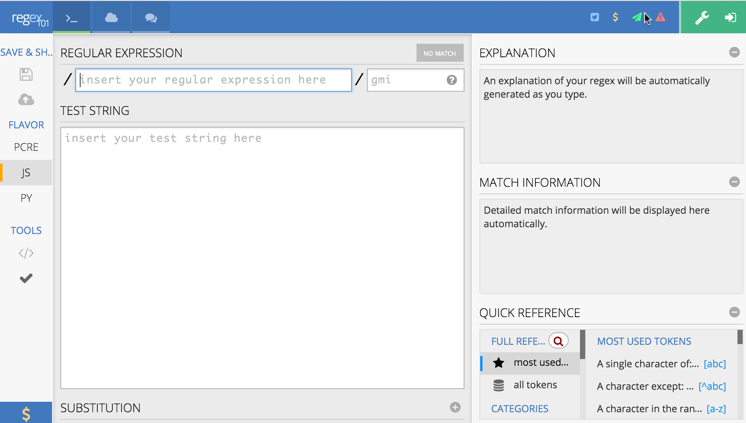# 肥客泉

## 知道这20个正则表达式，能让你少写1,000行代码

var reg = /^(\d{1,4})(-|\/)(\d{1,2})\2(\d{1,2})\$/; var r = fieldValue.match(reg);             if(r==null)alert('Date format error!'); 1 . 校验密码强度

^(?=.*\d)(?=.*[a-z])(?=.*[A-Z]).{8,10}\$ 2. 校验中文

^[\u4e00-\u9fa5]{0,}\$ 3. 由数字、26个英文字母或下划线组成的字符串^\w+\$ 4. 校验E-Mail 地址

[\w!#\$%&'*+/=?^_`{|}~-]+(?:\.[\w!#\$%&'*+/=?^_`{|}~-]+)*@(?:[\w](?:[\w-]*[\w])?\.)+[\w](?:[\w-]*[\w])? 5. 校验身份证号码

15位：

^[1-9]\d{7}((0\d)|(1[0-2]))(([0|1|2]\d)|3[0-1])\d{3}\$

18位：

^[1-9]\d{5}[1-9]\d{3}((0\d)|(1[0-2]))(([0|1|2]\d)|3[0-1])\d{3}([0-9]|X)\$ 6. 校验日期

“yyyy-mm-dd“ 格式的日期校验，已考虑平闰年。

^(?:(?!0000)[0-9]{4}-(?:(?:0[1-9]|1[0-2])-(?:0[1-9]|1[0-9]|2[0-8])|(?:0[13-9]|1[0-2])-(?:29|30)|(?:0|1)-31)|(?:[0-9]{2}(?:0||)|(?:0||)00)-02-29)\$ 7. 校验金额

^[0-9]+(.[0-9]{2})?\$ 8. 校验手机号

^(13[0-9]|14[5|7]|15[0|1|2|3|5|6|7|8|9]|18[0|1|2|3|5|6|7|8|9])\d{8}\$ 9. 判断IE的版本

IE目前还没被完全取代，很多页面还是需要做版本兼容，下面是IE版本检查的表达式。

^.*MSIE [5-8](?:\.[0-9]+)?(?!.*Trident\/[5-9]\.0).*\$ 10. 校验IP-v4地址

IP4 正则语句。

\b(?:(?:25[0-5]|2[0-4][0-9]|?[0-9][0-9]?)\.){3}(?:25[0-5]|2[0-4][0-9]|?[0-9][0-9]?)\b 11. 校验IP-v6地址

IP6 正则语句。

(([0-9a-fA-F]{1,4}:){7,7}[0-9a-fA-F]{1,4}|([0-9a-fA-F]{1,4}:){1,7}:|([0-9a-fA-F]{1,4}:){1,6}:[0-9a-fA-F]{1,4}|([0-9a-fA-F]{1,4}:){1,5}(:[0-9a-fA-F]{1,4}){1,2}|([0-9a-fA-F]{1,4}:){1,4}(:[0-9a-fA-F]{1,4}){1,3}|([0-9a-fA-F]{1,4}:){1,3}(:[0-9a-fA-F]{1,4}){1,4}|([0-9a-fA-F]{1,4}:){1,2}(:[0-9a-fA-F]{1,4}){1,5}|[0-9a-fA-F]{1,4}:((:[0-9a-fA-F]{1,4}){1,6})|:((:[0-9a-fA-F]{1,4}){1,7}|:)|fe80:(:[0-9a-fA-F]{0,4}){0,4}%[0-9a-zA-Z]{1,}|::(ffff(:0{1,4}){0,1}:){0,1}((25[0-5]|(2[0-4]|1{0,1}[0-9]){0,1}[0-9])\.){3,3}(25[0-5]|(2[0-4]|1{0,1}[0-9]){0,1}[0-9])|([0-9a-fA-F]{1,4}:){1,4}:((25[0-5]|(2[0-4]|1{0,1}[0-9]){0,1}[0-9])\.){3,3}(25[0-5]|(2[0-4]|1{0,1}[0-9]){0,1}[0-9])) 12. 检查URL的前缀

if (!s.match(/^[a-zA-Z]+:\/\//)){    s = 'http://' + s;} 13. 提取URL链接

^(f|ht){1}(tp|tps):\/\/([\w-]+\.)+[\w-]+(\/[\w- ./?%&=]*)? 14. 文件路径及扩展名校验

^([a-zA-Z]\:|\\)\\([^\\]+\\)*[^\/:*?"<>|]+\.txt(l)?\$ 15. 提取Color Hex Codes

^#([A-Fa-f0-9]{6}|[A-Fa-f0-9]{3})\$ 16. 提取网页图片

\< *[img][^\\>]*[src] *= *[\"\']{0,1}([^\"\'\ >]*) 17. 提取页面超链接

(<a\s*(?!.*\brel=)[^>]*)(href="https?:\/\/)((?!(?:(?:www\.)?'.implode('|(?:www\.)?', \$follow_list).'))[^"]+)"((?!.*\brel=)[^>]*)(?:[^>]*)> 18. 查找CSS属性

^\s*[a-zA-Z\-]+\s*[:]{1}\s[a-zA-Z0-9\s.#]+[;]{1} 19. 抽取注释

<!--(.*?)--> 20. 匹配HTML标签

<\/?\w+((\s+\w+(\s*=\s*(?:".*?"|'.*?'|[\^'">\s]+))?)+\s*|\s*)\/?> 正则表达式的相关语法学习正则表达式正则表达式在线测试工具

regex101 是一个非常不错的正则表达式在线测试工具，你可以直接在线测试你的正则表达式哦。